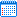2023-05-22       来源： 洁净园DR的冷负荷、热负荷计算

DR为一个净化空调系统，室内冷负荷Q=5.1kW，空调湿负荷d=0.29kg/h，门开和门闭时室内负荷变化忽略不计，万级洁净确定新风量定为800m³/h，门闭时送风量为2400m³/h，门开时送风量为3900m³/h（门开和闭合风量差值为1500立方米，可见围护结构气密性对节能的重要性）。

DR万级磁体室采用一次回风系统，新风处理到室内焓值，新风不承担室内负荷，新风空调箱仅承担新风负荷，室内循环空调箱承担全部室内冷负荷，循环空调箱在湿工况下工作，此种方式在工程设计中经常被采用。图所示为一次回风系统空气处理流程。DR万级

DR一次回风系统新风预冷量、温升值

Q新=

[1.2×G×(Iw-iL)]/3600=[1.2×800×（90.44-45.22）】/3600=12kW

PS: 1.2是空气在标准状态下的密度，单位为Kg/m³，G为新风量，焓值差

Q再=

[1.2×G×(io-iL')/3600=[1.2×2400×（38.85-35.9）/3600=2.36kW

DR净化空调箱制冷量Q=

=[1.2×G×(io-iL')]/3600=[1.2×2400×(45.22-5.9)]/3600=7.46kW

[1.2×G×(io-iL')]/3600=[1.2×3900×(41.3-36.06)]/3600=6.8kW

DR净化空调箱制冷量Q=

[1.2×G×(ic-iL'/)]/3600=[1.2×2400×(45.22-36.06)]/3600=11.9kW

Q新风=

[1.2×G×(iw-iL)]/3600=[1.2×1146×(90.44-45.22)]/3600=17.27kw

[1.2×G×（io-iL'）]/3600=[1.2×3735×（36.06-34.13）]/3600=2.4kw

R净化空调箱制冷量Q=

[1.2×G×(ic-iL')/3600=[1.2×3735×（4522-34.13)]/3600=13.8kW

OR百级手术室二次回风计算

OR百级手术室室内冷负荷Q=11.3kW，空调湿负荷d=0.6kg/h，门开和门闭时室内负荷变化忽略不计，百级手术新风量门开时定为1200m³/h，门闭时定为2400m³/h，手术室控制区2.6m×2.4m，手术室控制区送风量11000m³/h。OR I级手术二次回风系统i-d图

OR采用的是二次回风系统，从id图上分析（如上图），一次回风N点与处理后的新风风L点混合，经循环空调箱冷却处理到L'点，再与二次回风N混合至送风状态点O点。

Q=[1.2*G(iw-iL)/3600=[1.2*1200*(90.44-45.22)]/36O0=18KW

OR空调箱制冷量：

Q=[1.2*G*(ic-iL')1/3600=[1.2*11000*(45.22-35.73)]/3600=34.8kW

Q新=

[1.2*G*)iw-iL))]/3600=[1.2*2400*(90.44-45.22)]/3600=36kw

OR空调箱制冷量Q=

[1.2*G*(Ic-iL')]/3600 = [1.2*11000*(45.22-36.73)]/3600=34.8kW

Q再 =  [1.2*G*(Io-iL')]/3600 = [l.2*11000*(42.02-36.12)]/3600 = 21.6kW,如果OR采用一次回风，那么相对于DR其再热量要高出很多。